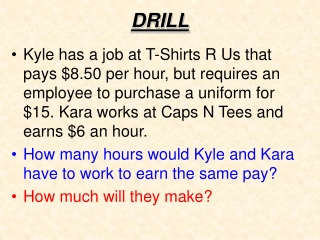DownloadDownload PresentationDRILL

# DRILL

Download Presentation## DRILL

- - - - - - - - - - - - - - - - - - - - - - - - - - - E N D - - - - - - - - - - - - - - - - - - - - - - - - - - -
##### Presentation Transcript

1. DRILL • Kyle has a job at T-Shirts R Us that pays \$8.50 per hour, but requires an employee to purchase a uniform for \$15. Kara works at Caps N Tees and earns \$6 an hour. • How many hours would Kyle and Kara have to work to earn the same pay? • How much will they make?

2. Up, Up, and Away • Two hot air balloons are in flight above a field. The first balloon is 20 meters above the ground and rising 25 meters per minute. The second balloon is 5 meters above the ground and rising 30 meters per minute. • When will the two balloons be at the same altitude?

3. Fill in the rest of the table by using each pattern and find after how many hours will they have earned the same amount of money?

4. Graphing on the Calculator Step 1:Type both equations into y = Step 2:Press Zoom Fit Step 3:*If you can not see the point of intersection press Zoom Out and Enter. Step 4:Press Stat  Calc  Intersect Step 5:Press Enter for First Curve, Second Curve, and Guess. (Press Enter 3 times)

5. Example • Construct a table for the following system of equations . • Graph the equations and find the point of intersection.

6. Parallel Lines • If two lines are parallel, then they do not intersect. (They have the same slope) • If two lines do not intersect then there is NO Solution to the system of equations.

7. Example y = mx + b m is the slope of the line

8. EXAMPLES

9. EXAMPLES